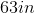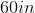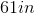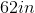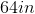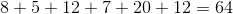ISEE Lower Level Quantitative : Plane Geometry

Example Questions

Example Question #64 : Geometry

What is the perimeter of the polygon below?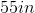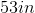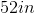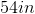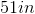Explanation:

To find the area of a perimeter, we add all of the side lengths together.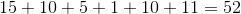Example Question #65 : Geometry

What is the perimeter of the polygon below?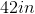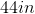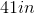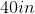Explanation:

To find the area of a perimeter, we add all of the side lengths together.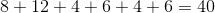Example Question #66 : Geometry

What is the perimeter of the polygon below?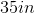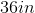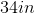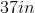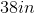Explanation:

To find the area of a perimeter, we add all of the side lengths together.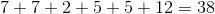Example Question #67 : Geometry

What is the perimeter of the polygon below?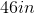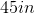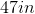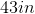Explanation:

To find the area of a perimeter, we add all of the side lengths together.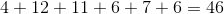Example Question #71 : Geometry

What is the perimeter of the polygon below?Explanation:

To find the area of a perimeter, we add all of the side lengths together.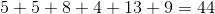Example Question #72 : Geometry

What is the perimeter of the polygon below?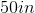Explanation:

To find the area of a perimeter, we add all of the side lengths together.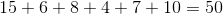Example Question #511 : Measurement & Data

What is the perimeter of the polygon below?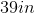Explanation:

To find the area of a perimeter, we add all of the side lengths together.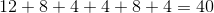Example Question #74 : Geometry

What is the perimeter of the polygon below?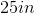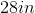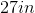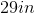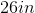Explanation:

To find the area of a perimeter, we add all of the side lengths together.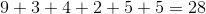Example Question #75 : Geometry

What is the perimeter of the polygon below?Explanation:

To find the area of a perimeter, we add all of the side lengths together.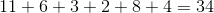Example Question #76 : Geometry

What is the perimeter of the polygon below?